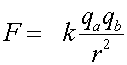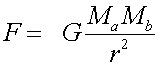Analogy between electric and gravitational forces

The electric force (Coulomb's Law) and gravitational forces have similar forms:One difference is that gravitational forces are always attractive, while the electric force can be either attractive (for opposite charges) or repulsive (for like charges).

Another difference is that the electric force is generally MUCH stronger:

k = 8.99x109 Nm2/C2      G = 6.67x10-11 Nm2/kg2

On the other hand, the gravitational force on a large body such as the entire earth can be much larger than the electrical force because the earth contains nearly equal numbers of electrons and protons and hence its total electric charge is small.